# Epsilon and delta

Karol

## Homework Statement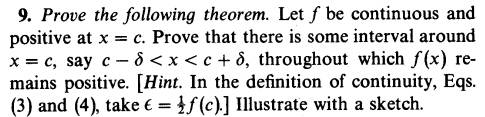## Homework Equations

Continuity:
$$\vert x-c \vert < \delta~\Rightarrow~\vert f(x)-f(c) \vert < \epsilon$$
$$\delta=\delta(c,\epsilon)$$

## The Attempt at a Solution

$$\vert f(x)-f(c) \vert <\frac{1}{2}f(c)~\Rightarrow~\vert x-c \vert < \delta_1$$
So i have this δ1 but what do i do with it?
And ε=½f(c) is big, maybe it will be in the negative zone.
Maybe i have to find a δ such that ##~\vert f(x)-f(c) \vert =0~##?
There is such a δ, so why was advised to take such a large ε?

Homework Helper
Gold Member
2022 Award

## The Attempt at a Solution

$$\vert f(x)-f(c) \vert <\frac{1}{2}f(c)~\Rightarrow~\vert x-c \vert < \delta_1$$
So i have this δ1 but what do i do with it?
And ε=½f(c) is big, maybe it will be in the negative zone.
Maybe i have to find a δ such that ##~\vert f(x)-f(c) \vert =0~##?
There is such a δ, so why was advised to take such a large ε?

You are still starting these proofs the wrong way round. Somehow you have to train yourself to stop writing things like:

$$\vert f(x)-f(c) \vert <\frac{1}{2}f(c)~\Rightarrow~\vert x-c \vert < \delta_1$$

You must, must, must stop yourself from doing this.

For this problem I would first try to "prove" it using a graph of the function and a geometric argument.

Homework Helper
Gold Member
2022 Award

## Homework Equations

Continuity:
$$\vert x-c \vert < \delta~\Rightarrow~\vert f(x)-f(c) \vert < \epsilon$$

The above is continuity.

## The Attempt at a Solution

$$\vert f(x)-f(c) \vert <\frac{1}{2}f(c)~\Rightarrow~\vert x-c \vert < \delta_1$$

And this is what you write. You must see the difference. Every time you turn it round the wrong way.

Karol
I was wrong at the definition of continuity:
$$\vert f(x)-f(c) \vert < \epsilon~\Rightarrow~\vert x-c \vert < \delta$$
The ε is to the intersection with x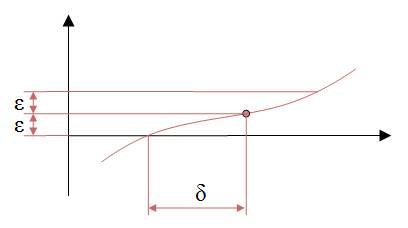Mentor
I was wrong at the definition of continuity:
$$\vert f(x)-f(c) \vert < \epsilon~\Rightarrow~\vert x-c \vert < \delta$$
No, what you have above is backwards. The implication you showed in post 1 has the implication in the right order.
In words, "If x is close to c, then f(x) will be close to f(c)"
The delta and epsilon quantify the "close to" terms.
Karol said:
The ε is to the intersection with x
??
Karol said:
In your drawing, where is x? Where is c? Is the circled point on the curve (c, f(c))?

Homework Helper
Gold Member
2022 Award
I was wrong at the definition of continuity:
$$\vert f(x)-f(c) \vert < \epsilon~\Rightarrow~\vert x-c \vert < \delta$$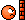•SammyS
Dr.D
I had a friend, a fraternity brother actually, who always said that his ambition in life was to be an epsilon and delta picker.

•PeroK
Karol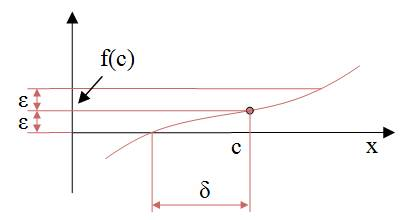Karol
If f(c)>0 i can take ε small and it will still be ##~\vert f(x)-f(c) \vert >0~## and find a δ because of continuity, so why do i need the ##~\epsilon=\frac{1}{2}f(c)~##?

Mentor
In your drawing in post #8, you have ##\epsilon = f(c)##, which isn't what the hint is saying.

Karol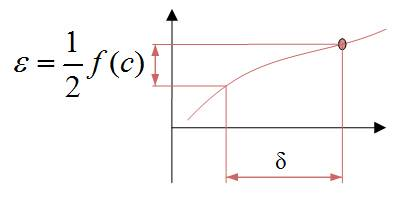Thank you Mark, Dr.D and PeroK

Mentor
@Karol, did you actually prove the theorem? The problem asks you to prove that statement, and illustrate with a sketch.

Karol
Because of continuity i can find a δ for ##~\epsilon=\frac{1}{2}f(c)~##, so in this interval: ##~c-\delta<x<c+\delta~##, f(x)>0